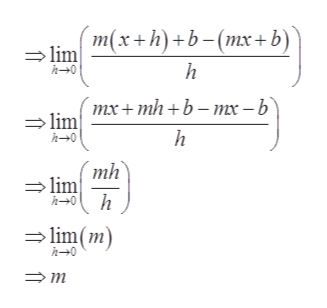# Find the deriviate of the function using the definition of a derivative. State the domain of the function and the domain of its derivative. f(x)=mx+b

Question
1 views

Find the deriviate of the function using the definition of a derivative. State the domain of the function and the domain of its derivative.

f(x)=mx+b

check_circle

Step 1

Consider the given function.

Step 2

Formula used:

The definition of a derivative is given by:

Step 3

Apply the above ...help_outlineImage Transcriptionclosem(x+h)+b-(mx+b) lim h h0 тх + mh +b - mх- b lim h0 h mh lim h h0 lim(m) h0 fullscreen

### Want to see the full answer?

See Solution

#### Want to see this answer and more?

Solutions are written by subject experts who are available 24/7. Questions are typically answered within 1 hour.*

See Solution
*Response times may vary by subject and question.
Tagged in

### Calculus